Next: Cross Ratios and Projective Up: Cross-Ratios on the Projective Previous: Cross-Ratios on the Projective

#### Cross Ratios and Length Ratios:

In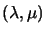coordinates on the line MN, N is represented by (0, 1) and serves as the origin'' and M is represented by (1, 0) and serves as the point at infinity''. For an arbitrary point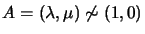, we can rescaleto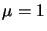, and represent A by its affine coordinates''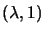, or just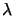for short. Since we have mapped M to infinity, this is just linear distance along the line from N. Hence, setting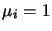in 3.1, the cross ratio becomes a ratio of length ratios. The ancient Greek mathematicians already used cross ratios in this form.

Exercise 3.2   : Let D be the point at infinity on the projective line, and let A, B, C be three finite points. Show that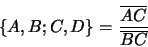Exercise 3.3   If MN is the line between two points M and N in the projective plane, use theparameterization and the results of section 2.2.1 to show that the cross ratio of four points A,B,C,D on the line is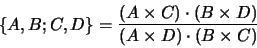Next: Cross Ratios and Projective Up: Cross-Ratios on the Projective Previous: Cross-Ratios on the Projective
Bill Triggs
1998-11-13